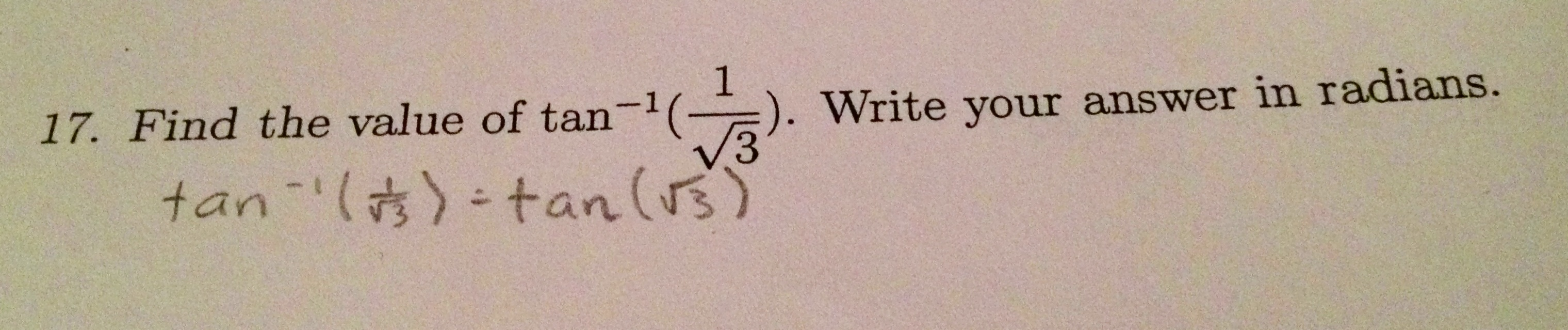Categories

# Inverse Trig FunctionsHow would you help these students?

Thanks to Tina Cardone for the submissions. Head over to the Productive Struggle blog for further analysis and discussion of these questions.

## 4 replies on “Inverse Trig Functions”

#1: I just don’t know. I’ve had a couple of students for a long time, several semesters, and with whom I’ve had many conversations about problems involving inverse functions, including discussion that the “-1” notation does not always indicate a reciprocal. They’re pretty good at math in general. And they still jump to reciprocals whenever we first look at a problem involving inverse trig functions. It just won’t stick, and I cannot explain it. I suppose switching to the arctan notation is a potential solution.

In this problem, the student might recognize that there is an issue if he/she reads the “write your answer in radians”. Some thought should indicate that tan(sqrt(3)) is usually not considered to be a number in radians, but rather that the input to tangent is.

#2 and #3 — both compute (sqrt(3)/2)/(1/2). It’s a tricky problem — these students have been inculcated with the unit circle. There’s a unit circle value staring at them in the problem, so they expect the answer to involve a simple computation off of the unit circle as a result. They seem to know the unit circle well enough to recognize sqrt(3)/2 as sin(pi/3) and 1/2 as cos(pi/3). It looks like the second one decided to double pi/3 to pi/6 because (sqrt(3)/2)/(1/2) works out to twice sqrt(3)/2. We don’t know if they’ve been taught the “draw a triangle” trick to compute these things, but perhaps with a weirder value, like sqrt{7}/3, inside, it would occur to them. If you have the fabulous problem that your students know their sines and cosines on the unit circle too well, I might start pushing more problems that deal with weirder values, like unit circle tangents or secants. Fun values also include cos(2pi/5) = (-1 + sqrt{5})/4, cos(pi/8) = Sqrt(2+Sqrt(2))/4, and tan(pi/12) = 2 – sqrt(3).

They did learn to draw the triangle. I like the phrase “inculcated with the unit circle” and you’re exactly right, I taught them that the unit circle is their best friend and now they turn to it every time. We did usually use stranger values when they needed to turn to a triangle in class so that was a misleading question to put on an exam.

Switching to arctan notation is a good idea for a small improvement. That might help the first student some.

I think the bigger advice I’d give all these kids is to draw a triangle, every time. The last one at least got to realizing that an inverse tangent is telling you something about an angle, but there’s the additional step after that of drawing the triangle (or unit circle) to illustrate the angle whose tangent is sqrt(3)/2.

[…] Feel free to check out the exact mistakes my students made at the Math Mistakes blog. […]